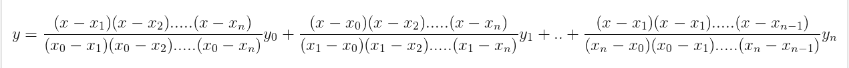# Lagrange Interpolation Formula

Lagrange polynomials are used for polynomial interpolation. For a given set of distinct points

$$\begin{array}{l}x_{j}\end{array}$$
and numbers
$$\begin{array}{l}y_{j}\end{array}$$
. Lagrange’s interpolation is also an
$$\begin{array}{l}n^{th}\end{array}$$
degree polynomial approximation to f(x).

Find the Lagrange Interpolation Formula given below,### Solved Example

Question:

Find the value of y at x = 0 given some set of values (-2, 5), (1, 7), (3, 11), (7, 34).

Solution:

Given the known values are,

x = 0 ; x0 = -2 ; x1 = 1 ; x= 3 ; x3 = 7 ; y0 = 5 ; y1 = 7 ; y2 = 11 ; y3 = 34

Using the interpolation formula,

y =

$$\begin{array}{l}\frac{(x-x_{1})(x-x_{2})…..(x-x_{n})}{(x_{0}-x_{1})(x_{0}-x_{2})…..(x_{0}-x_{n})}\end{array}$$
$$\begin{array}{l}y_{0}\end{array}$$
+
$$\begin{array}{l}\frac{(x-x_{0})(x-x_{2})…..(x-x_{n})}{(x_{1}-x_{0})(x_{1}-x_{2})…..(x_{1}-x_{n})}\end{array}$$
$$\begin{array}{l}y_{1}\end{array}$$
+ …. +
$$\begin{array}{l}\frac{(x-x_{1})(x-x_{1})…..(x-x_{n-1})}{(x_{n}-x_{0})(x_{0}-x_{1})…..(x_{n}-x_{n-1})}\end{array}$$
$$\begin{array}{l}y_{n}\end{array}$$

y =

$$\begin{array}{l}\frac{(0-1)(0-3)(0-7)}{(-2-1)(-2-3)(-2-7)}\times 5\end{array}$$
+
$$\begin{array}{l}\frac{(0+2)(0-3)(0-7)}{(1+2)(1-3)(1-7)}\times 7\end{array}$$
+
$$\begin{array}{l}\frac{(0+2)(0-1)(0-7)}{(3+2)(3-1)(3-7)}\times 11\end{array}$$
+
$$\begin{array}{l}\frac{(0+2)(0-1)(0-3)}{(7+2)(7-1)(7-3)}\times 34\end{array}$$

y =

$$\begin{array}{l}\frac{21}{27}\end{array}$$
+
$$\begin{array}{l}\frac{49}{6}\end{array}$$
+
$$\begin{array}{l}\frac{-77}{20}\end{array}$$
+
$$\begin{array}{l}\frac{51}{54}\end{array}$$

y =

$$\begin{array}{l}\frac{1087}{180}\end{array}$$